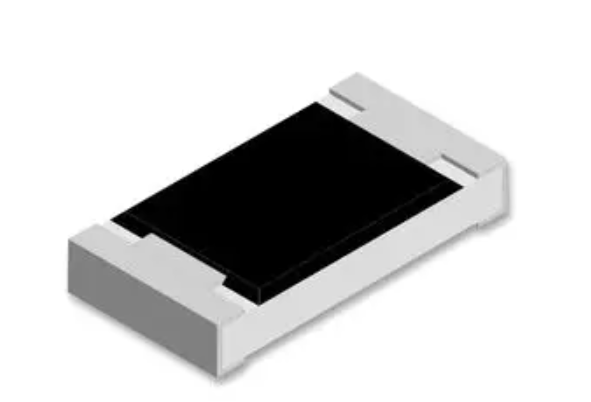< img src="https://mc.yandex.ru/watch/53493796" style="position:absolute; left:-9999px;" alt="" />
Need Help?## CRGCQ0805J150K

The CRGCQ0805J150K is a general-purpose resistor. It is a surface-mount chip resistor with a 0805 package size, which measures 2.0mm x 1.25mm. The “J” in the part number indicates that the resistor has a tolerance of 5%. The “150K” indicates the resistance value, which is 150 kilohms. This resistor is commonly used in a variety […]

## Description

The CRGCQ0805J150K is a general-purpose resistor. It is a surface-mount chip resistor with a 0805 package size, which measures 2.0mm x 1.25mm. The “J” in the part number indicates that the resistor has a tolerance of 5%. The “150K” indicates the resistance value, which is 150 kilohms.

This resistor is commonly used in a variety of electronic circuits and applications, including power supplies, amplifiers, and digital circuits. Its small size and surface-mount package make it easy to use in compact designs.

It’s important to note that the CRGCQ0805J150K is just one example of a general-purpose resistor, and there are many other resistor types and values available for different applications. When selecting a resistor for a specific application, it’s important to consider factors such as the required resistance value, power rating, tolerance, and temperature coefficient.

## CRGCQ0805J150K Feature

• Size: 0805 (metric) or 2012 (imperial)
• Resistance value: 15 ohms (15000 milliohms)
• Tolerance: 5%
• Power rating: 0.125 watts
• Temperature coefficient of resistance (TCR): ±200 ppm/°C
• Operating temperature range: -55°C to +155°C
• Maximum working voltage: 150V

### FAQ

Q: What is the resistor?

A: The resistor is a general-purpose surface-mount chip resistor.

Q: What is the size of the resistor?

A: The resistor is a standard 0805 package size, which measures approximately 2mm x 1.25mm.

Q: What is the power rating of the resistor?

A: The resistor has a power rating of 1/8 Watt (0.125W).

Q: What is the resistance value of CRGCQ0805J150K?

A: The resistance value of the resistor is 15 ohms.

Q: What is the tolerance of CRGCQ0805J150K?

A: CRGCQ0805J150K has a tolerance of 5%.

SEND EMAIL TO US